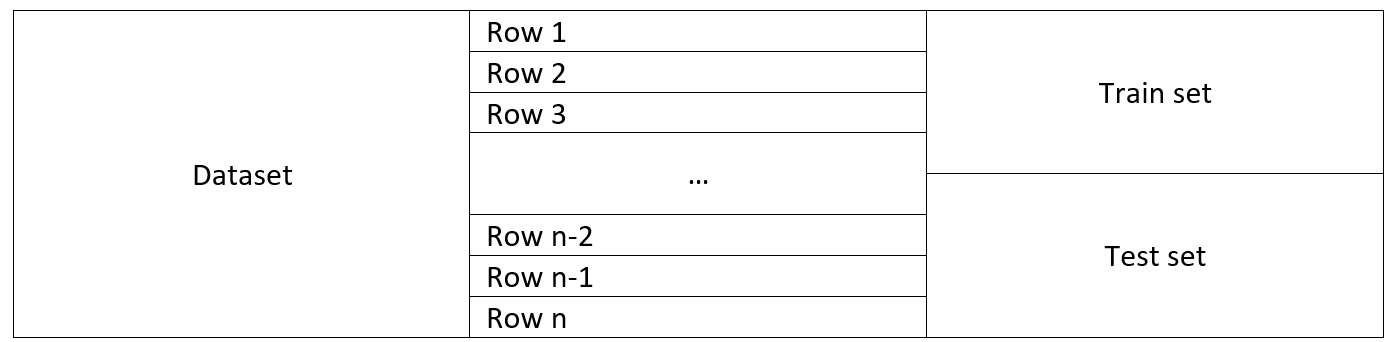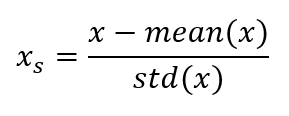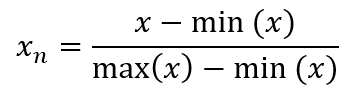# .css-4zleql{display:block;}kristijan-pajtasev# Machine Learning - Data preprocessing

Subscribe to my newsletter and never miss my upcoming articles

As part of my trying to complete Machine Learning A-Z Udemy course, this series of posts, starting with this one, will contain note I gather from it.

### Dependent vs independent variables

• Dependent – variable being tested and measured – predicted result
• Independent – variable being changed or controlled – features

Used libraries(python):

• numpy, a library containing mathematical tools
• matplotlib.pyplot, plotting library
• pandas – importing datasets
• sklearn.preprocessing – library for processing data

Importing dataset with pandas:

``````import pandas as pd
``````

### Missing data

Option 1:

• remove rows with missing data
• dangerous because we might be losing valuable information Option 2:
• set missing values to mean of that feature

Library used:

``````sklearn.preprocessing.Imputer
``````

### Categorical data

Labels need to be converted into numbers - Euclidean distance can’t be calculated on labels Library:

``````sklearn.preprocessing.LabelEncoder
``````

Problem with LabelEncoder: converting labels into numbers can lead to problems as numbers can be ordered. Labels not necessary Solution: Creating feature per label Library: sklearn.preprocessing.OneHotEncoder

### Splitting data

For creating a model, data needs to be split into two sets, train and test. The train set is the one we use for creating a model, and the test is one we use to evaluate that mode's correctness. Library: sklearn.model_selection.train_test_split Usual ration: 70-80% for train data### Feature scaling

One feature, because of large values, can dominate the smaller number value feature. This is why all features should be scaled to the same scale. Option 1, standardization: Each value is reduced by the mean and divided by the standard deviation.Option 2, normalization: Reduce each x by minimal x value. Ather that, divide by the difference between the maximum and minimum value of x.Library:

``````sklearn.preprocessing.StandardScaler
``````